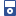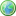Accessibility options:

# Trigonometry resources

Show me all resources applicable to

### iPOD Video (1)Trigonometric ratios for any angle - an Animation
Video for iPod. This resource is released under a Creative Commons license Attribution-Non-Commercial-No Derivative Works and the copyright is held by Skillbank Solutions Ltd.

### Teach Yourself (11)a cos x +b sin x = Rcos(x-a)
In this unit we explore how the sum of two trigonometric functions can be expressed as a single trigonometric function.Cosecant, Secant and Cotangent
This unit explains what is meant by these three trigonometric ratios. Graphs of the corresponding functions are provided.Pythagoras' theorem
This unit states Pythagoras' theorem and illustrates its use in the solution of two- and three-dimensional problems.Radians
This unit looks at how angles can be measured in radians.Sine, cosine and tangent of an angle of any size
This unit explains how we define the trig ratios sine, cosine, and tangent for an angle of arbitrary size.The addition formulae
This unit details the addition formulae for sin(A+B), sin(A-B), etc.The double angle formulae
This unit looks at the trigonometric formulae known as the double angle formulae.Triangle formulae
This unit explains the sine rule, cosine rule and formula for finding the area of a triangle.Trigonometric equations
This workbook explains how a number of trigonometric equations can be solved by making reference to a Table of standard results and using the symmetries and periodicities present in the graphs of trig functions.Trigonometric identities
In this unit several identities are derived and then used in the solution of trigonometric equations.Trigonometrical ratios in a right angled triangle
This unit introduces the ratios sine, cosine and tangent, with reference to a right angled triangle.

### Test Yourself (1)Maths EG
Computer-aided assessment of maths, stats and numeracy from GCSE to undergraduate level 2. These resources have been made available under a Creative Common licence by Martin Greenhow and Abdulrahman Kamavi, Brunel University.

### Video (12)Pythagoras' theorem
Pythagoras theorem - the square on the hypotenuse is equal to the sum of the squares on the other two sides - is well known. In this tutorial we revise the theorem and use it to solve problems in right-angled triangles. A less familiar form of the theorem is also considered. (Mathtutor Video Tutorial) This resource is released under a Creative Commons license Attribution-Non-Commercial-No Derivative Works and the copyright is held by Skillbank Solutions Ltd.Pythagoras' Theorem - an Animation
This mathtutor animation shows visually that the square on the hypotenuse is equal to the sum of the squares on the other two sides. This resource is released under a Creative Commons license Attribution-Non-Commercial-No Derivative Works and the copyright is held by Skillbank Solutions Ltd.Radian measure
Most people usually learn to measure an angle in degrees. But in many scientific and engineering calculations radians are used in preference to degrees. (Mathtutor Video Tutorial) This resource is released under a Creative Commons license Attribution-Non-Commercial-No Derivative Works and the copyright is held by Skillbank Solutions Ltd.Sine, cosine and tangent of an angle of any size
Knowledge of the trigonometric ratios sine, cosine and tangent is vital in many fields of engineering, maths and science. This unit explains how the sine, cosine and tangent of an arbitrarily sized angle can be found. (Mathtutor Video Tutorial) This resource is released under a Creative Commons license Attribution-Non-Commercial-No Derivative Works and the copyright is held by Skillbank Solutions Ltd.Solving trigonometrical equations
The strategy we adopt in solving trigonometric equations is to find one solution using knowledge of commonly occurring angles and then use the symmetries in the graphs of the trigonometric functions to deduce additional solutions. (Mathtutor Video Tutorial) This resource is released under a Creative Commons license Attribution-Non-Commercial-No Derivative Works and the copyright is held by Skillbank Solutions Ltd.The addition formulae
There are six so-called addition formulae often needed in the solution of trigonometric problems. In this unit we start with one and derive a second. Then we take another one as given and derive a second one from that. Finally we use these four to help us derive the final two. (Mathtutor Video Tutorial)The double angle formulae
Double angle formulae are so called because they involve trigonometric functions of double angles e.g. sin 2A, cos 2A and tan 2A. (Mathtutor Video Tutorial) This resource is released under a Creative Commons license Attribution-Non-Commercial-No Derivative Works and the copyright is held by Skillbank Solutions Ltd.The form Rcos(x-a)
In this unit we explore how the sum of two trigonometric functions e.g.3 cos x plus 4 sin x, can be expressed as a single trigonometric function. Having the ability to do this enables us to solve trigonometric equations and find maximum and minimum values. (Mathtutor Video Tutorial) This resource is released under a Creative Commons license Attribution-Non-Commercial-No Derivative Works and the copyright is held by Skillbank Solutions Ltd.Triangle formulae
A common mathematical problem is to find the angles or lengths of the sides of a triangle when some, but not all, of these quantities are known. It is also useful to be able to calculate the area of a triangle from some of this information. (Mathtutor Video Tutorial) This resource is released under a Creative Commons license Attribution-Non-Commercial-No Derivative Works and the copyright is held by Skillbank Solutions Ltd.Trig functions: cosecant, secant and cotangent
In this unit we see how the three trigonometric ratios cosecant, secant and cotangent can appear in trigonometric identities and in the solution of trigonometric equations. Graphs of the functions are obtained from a knowledge of sine, cosine and tangent. (Mathtutor Video Tutorial) This resource is released under a Creative Commons license Attribution-Non-Commercial-No Derivative Works and the copyright is held by Skillbank Solutions Ltd.Trig ratios in a right angled triangle
Knowledge of the trigonometric ratios of sine, cosine and tangent is vital in very many fields of engineering, science and maths. This unit introduces them and provides examples of how they can be used to solve problems. (Mathtutor Video Tutorial) This resource is released under a Creative Commons license Attribution-Non-Commercial-No Derivative Works and the copyright is held by Skillbank Solutions Ltd.Trigonometric identities
In this unit we consider trigonometric identities and how to use them to solve trigonometric equations. (Mathtutor Video Tutorial) This resource is released under a Creative Commons license Attribution-Non-Commercial-No Derivative Works and the copyright is held by Skillbank Solutions Ltd.

### Video with captions which require edits (12)Pythagoras' theorem
Pythagoras theorem - the square on the hypotenuse is equal to the sum of the squares on the other two sides - is well known. In this tutorial we revise the theorem and use it to solve problems in right-angled triangles. A less familiar form of the theorem is also considered. (Mathtutor Video Tutorial) The video is released under a Creative Commons license Attribution-Non-Commercial-No Derivative Works and the copyright is held by Skillbank Solutions Ltd.Pythagoras' Theorem - an Animation
This mathtutor animation shows visually that the square on the hypotenuse is equal to the sum of the squares on the other two sides. The video is released under a Creative Commons license Attribution-Non-Commercial-No Derivative Works and the copyright is held by Skillbank Solutions Ltd.Radian measure
Most people usually learn to measure an angle in degrees. But in many scientific and engineering calculations radians are used in preference to degrees. (Mathtutor Video Tutorial) The video is released under a Creative Commons license Attribution-Non-Commercial-No Derivative Works and the copyright is held by Skillbank Solutions Ltd.Sine, cosine and tangent of an angle of any size
Knowledge of the trigonometric ratios sine, cosine and tangent is vital in many fields of engineering, maths and science. This unit explains how the sine, cosine and tangent of an arbitrarily sized angle can be found. (Mathtutor Video Tutorial) The video is released under a Creative Commons license Attribution-Non-Commercial-No Derivative Works and the copyright is held by Skillbank Solutions Ltd.Solving trigonometrical equations
The strategy we adopt in solving trigonometric equations is to find one solution using knowledge of commonly occurring angles and then use the symmetries in the graphs of the trigonometric functions to deduce additional solutions. (Mathtutor Video Tutorial) The video is released under a Creative Commons license Attribution-Non-Commercial-No Derivative Works and the copyright is held by Skillbank Solutions Ltd.The addition formulae
There are six so-called addition formulae often needed in the solution of trigonometric problems. In this unit we start with one and derive a second. Then we take another one as given and derive a second one from that. Finally we use these four to help us derive the final two. (Mathtutor Video Tutorial)The double angle formulae
Double angle formulae are so called because they involve trigonometric functions of double angles e.g. sin 2A, cos 2A and tan 2A. (Mathtutor Video Tutorial) The video is released under a Creative Commons license Attribution-Non-Commercial-No Derivative Works and the copyright is held by Skillbank Solutions Ltd.The form Rcos(x-a)
In this unit we explore how the sum of two trigonometric functions e.g.3 cos x plus 4 sin x, can be expressed as a single trigonometric function. Having the ability to do this enables us to solve trigonometric equations and find maximum and minimum values. (Mathtutor Video Tutorial) The video is released under a Creative Commons license Attribution-Non-Commercial-No Derivative Works and the copyright is held by Skillbank Solutions Ltd.Triangle formulae
A common mathematical problem is to find the angles or lengths of the sides of a triangle when some, but not all, of these quantities are known. It is also useful to be able to calculate the area of a triangle from some of this information. (Mathtutor Video Tutorial) The video is released under a Creative Commons license Attribution-Non-Commercial-No Derivative Works and the copyright is held by Skillbank Solutions Ltd.Trig functions: cosecant, secant and cotangent
In this unit we see how the three trigonometric ratios cosecant, secant and cotangent can appear in trigonometric identities and in the solution of trigonometric equations. Graphs of the functions are obtained from a knowledge of sine, cosine and tangent. (Mathtutor Video Tutorial) The video is released under a Creative Commons license Attribution-Non-Commercial-No Derivative Works and the copyright is held by Skillbank Solutions Ltd.Trig ratios in a right angled triangle
Knowledge of the trigonometric ratios of sine, cosine and tangent is vital in very many fields of engineering, science and maths. This unit introduces them and provides examples of how they can be used to solve problems. (Mathtutor Video Tutorial) The video is released under a Creative Commons license Attribution-Non-Commercial-No Derivative Works and the copyright is held by Skillbank Solutions Ltd.Trigonometric identities
In this unit we consider trigonometric identities and how to use them to solve trigonometric equations. (Mathtutor Video Tutorial) The video is released under a Creative Commons license Attribution-Non-Commercial-No Derivative Works and the copyright is held by Skillbank Solutions Ltd.

Website design by Pink Mayhem, Leicester